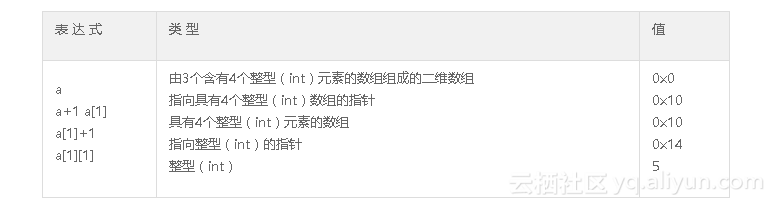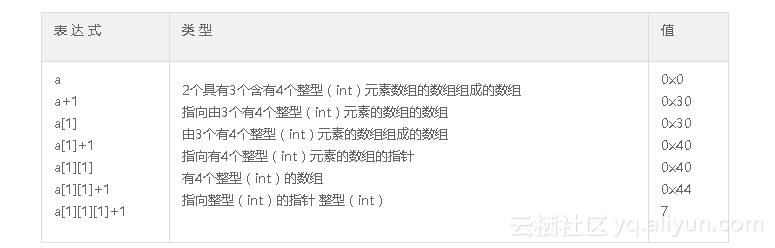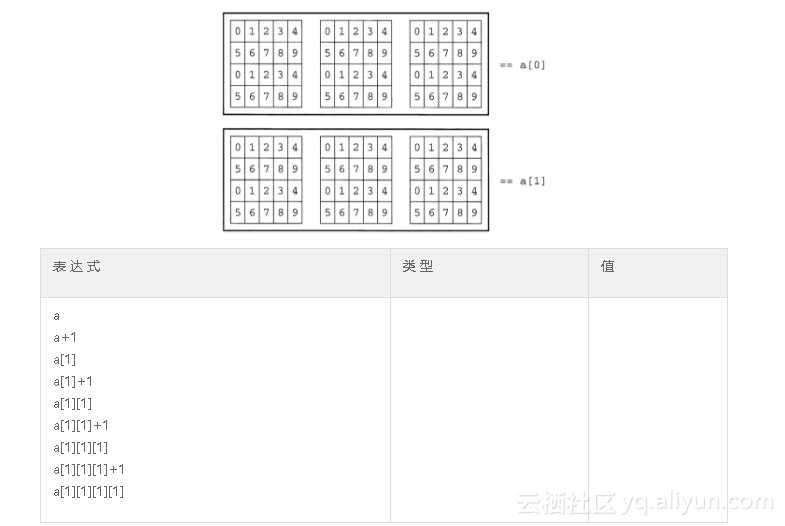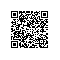# 《C和C++代码精粹》——2.11　更高深的内容

### 2.11　更高深的内容

C和C++代码精粹

int a  ={{{0,1,2,3},{4,5,6,7},{8,9,0,1}},
{{2,3,4,5},{6,7,8,9},{0,1,2,3}}};int (*p)   = a;// array9.c:    使用一个二维数组指针
#include <iostream>
using namespace std;

main()
{
int a[] = {{{0,1,2,3},{4,5,6,7},{8,9,0,1}},
{{2,3,4,5},{6,7,8,9},{0,1,2,3}}};
int (*p) = a;
size_t ntables = sizeof a / sizeof a;
size_t nrows   = sizeof a / sizeof a;
size_t ncols   = sizeof a / sizeof a;

cout << "sizeof(*p) == " << sizeof *p << endl;
cout << "sizeof(a) == " << sizeof a << endl;
for (int i = 0; i < ntables; ++i)
{
for (int j = 0; j < nrows; ++j)
{
for (int k = 0; k < ncols; ++k)
cout << p[i][j][k] << ' ';
cout << endl;
}
cout << endl;
}
}

//输出:
sizeof(*p) == 48
sizeof(a) == 16
0 1 2 3
4 5 6 7
8 9 0 1

2 3 4 5
6 7 8 9
0 1 2 3sizeof  a /sizeof  a


sizeof  a /sizeof  a


sizeof  a /sizeof  a


sizeof  a /sizeof  a


sizeof  a /sizeof  a


sizeof  a… /sizeof  a…
{_n_-_1__subscripts_}       {_n_-_1__subscripts_}


int  a =
{
{
{ {0,1,2,3,4},{5,6,7,8,9},{0,1,2,3,4},{5,6,7,8,9} },
{ {0,1,2,3,4},{5,6,7,8,9},{0,1,2,3,4},{5,6,7,8,9} }
{ {0,1,2,3,4},{5,6,7,8,9},{0,1,2,3,4},{5,6,7,8,9} }
},
{
{ {0,1,2,3,4},{5,6,7,8,9},{0,1,2,3,4},{5,6,7,8,9} },
{ {0,1,2,3,4},{5,6,7,8,9},{0,1,2,3,4},{5,6,7,8,9} }
{ {0,1,2,3,4},{5,6,7,8,9},{0,1,2,3,4},{5,6,7,8,9} }
}
};使用钉钉扫一扫加入圈子
+ 订阅